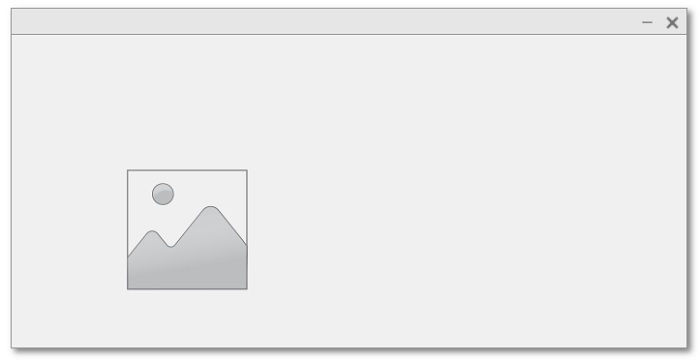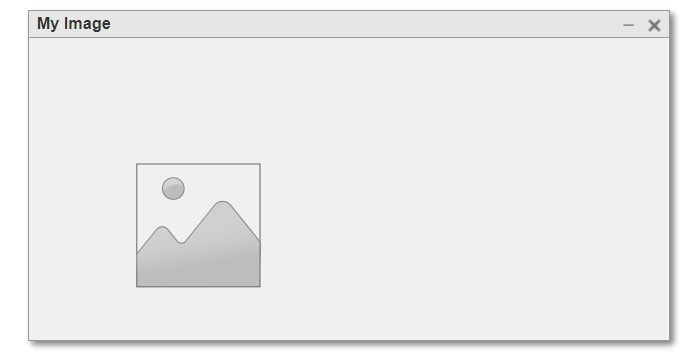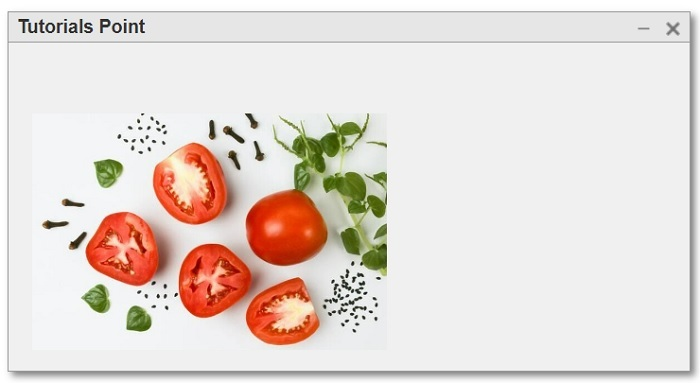# How to Create an Image Component in MATLAB?

In MATLAB, we can create various types of GUI components like EditField, buttons, hyperlink, image, etc. In this tutorial, I will explain how can you create an image component in MATLAB programming. But before that let us first get an overview of image component in MATLAB.

## What is Image Component in MATLAB?

In MATLAB, Image Component is a GUI (Graphical User Interface) component used to show an image in a MATLAB application. This component is widely used in applications where it is required to handle images like in image processing and analysis.

To create an image component, MATLAB provides a built-in function 'uiimage' which is basically a part of MATLAB App Designer. Using this function, we can easily control the behavior and appearance of an image displayed in a user interface.

Now, let us discuss the step-by-step procedure to create an image component in MATLAB.

## How to Create Image Component in MATLAB?

The stepwise process for creating an image component is explain here:

Step (1) – Read the input image that you want to display in the image component. For this, you can use the 'imread' function.

### Syntax

I = imread('image.jpg');


Step (2) – Process your image if required. For example, convert the input image from RGB to gray scale. It is optional step, so you may skip.

Step (3) – Create the image component using the 'uiimage' function.

### Syntax

img = uiimage('ImageSource', 'image.jpg');


In MATLAB, you can create an image component by following these three simple steps.

Now, let us consider different syntax formats of the 'uiimage' component to create an image component.

## Create a Simple Image Component

We can use the following syntax of the 'uiimage' function to create an image component in MATLAB with default properties:

img = uiimage();


### Example

The following example demonstrates the code implementation for this syntax.

% MATLAB program to create simple image component
% Create a simple image component
img = uiimage();


### Output## Create an Image Component withing Specified Container

The following syntax of the 'uiimage' function is used to create an image component within a specified container:

img = uiimage(parent);


Here, 'parent' is the container where the image component will be placed. It could be a 'uifigure' or 'uipanel'.

### Example

Consider the following example to understand the code implementation for this syntax:

% MATLAB program to create an image component with parent container
% Create a panel as parent container
fig = uifigure('Name', 'My Image');

% Create the image component
img = uiimage(fig);


### Output## Create an Image Component with Specified Properties

We can use the following syntax of the 'uiimage' function to create an image component with specified properties:

img = uiimage(___, Name, Value);


Here, the name-value pair is used to customize the properties of the image component.

### Example

Let us now take an example to understand the code implementation for this syntax.

% MATLAB program to create an image component with custom properties

% Create a parent container
fig = uifigure('Name', 'Tutorials Point');

% Create the image component with custom properties
img = uiimage(fig, 'Position', [25 25 250 175], 'ImageSource', I);


### Output## Conclusion

This is all about creating an image component in MATLAB. In conclusion, MATLAB has a built-in function 'uiimage' which is used to create a GUI image component in MATLAB application. In this tutorial, I explained what is an image component and how can you create an image component MATLAB application. I also included some example programs for better clarity of the concept.

Updated on: 07-Sep-2023

32 Views